Author: Oscar Cronquist Article last updated on March 25, 2023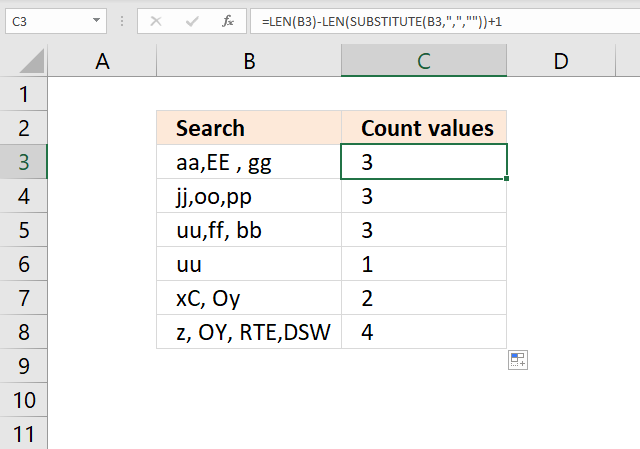This article demonstrates formulas that count values in cell based on a delimiting character. The image above shows a formula in cell C3 that counts strings between a given character in a specific cell.

## 1. How to count comma-separated values in a cell?The following formula counts the number of strings in a single cell using a comma as a delimiting character.

Formula in cell C3:

=LEN(B3)-LEN(SUBSTITUTE(B3,",",""))+1

### Explaining formula in cell C3

#### Step 1 - Substitute comma with nothing

The SUBSTITUTE function replaces a specific text string in a value.

SUBSTITUTE(textold_textnew_text, [instance_num])

SUBSTITUTE(B3,",","")

becomes

SUBSTITUTE("aa,EE , gg",",","")

and returns "aaEE gg".

#### Step 2 - Count characters in the substituted text

The LEN function counts the number of characters in a string.

LEN(SUBSTITUTE(B3,",",""))

becomes

LEN("aaEE gg")

and returns 8.

#### Step 3 - Count characters in the original text

LEN(B3)

becomes

LEN("aa,EE , gg")

and returns 10.

#### Step 4 - Subtract original text length with substituted text length

LEN(B3)-LEN(SUBSTITUTE(B3,",",""))+1

becomes

10-8+1 equals 3.

## 2. How to count comma-separated values in a cell range?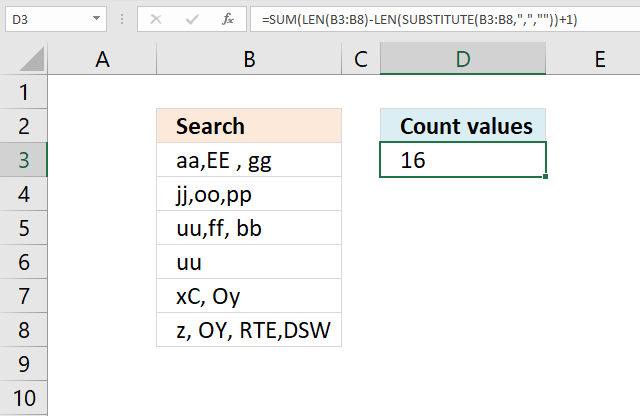Array formula in cell C3:

=SUM(LEN(B3:B8)-LEN(SUBSTITUTE(B3:B8, ",", ""))+1)

### How to enter an array formula

Enter the formula as a regular formula if you use Excel 365, follow these steps if you use an older version.

1. Copy above formula.
2. Double press with left mouse button on cell C3.
3. Paste to cell C3
4. Press and hold CTRL + SHIFT keys simultaneously.
5. Press Enter once.
6. Release all keys.

The formula will now look like this: {=SUM(LEN(B3:B8)-LEN(SUBSTITUTE(B3:B8, ",", ""))+1)}

Don't enter these characters yourself, they appear automatically.

### Explaining formula in cell C3

#### Step 1 - Substitute comma with nothing

The SUBSTITUTE function replaces a specific text string in a value.

SUBSTITUTE(textold_textnew_text, [instance_num])

SUBSTITUTE(B3:B8, ",", "")

becomes

SUBSTITUTE({"aa | EE | gg";"jj | oo | pp";"uu | ff | bb";"uu";"xC | Oy";"z | OY | RTE | DSW"},",","")

and returns

{"aaEE gg";"jjoopp";"uuff bb";"uu";"xC Oy";"z OY RTEDSW"}.

#### Step 2 - Count characters in the substituted text

The LEN function counts the number of characters in a string.

LEN(SUBSTITUTE(B3:B8, ",", ""))

becomes

LEN({"aaEE gg";"jjoopp";"uuff bb";"uu";"xC Oy";"z OY RTEDSW"})

and returns {8; 6; 7; 2; 5; 11}.

#### Step 3 - Count characters in the original text

LEN(B3:B8)

becomes

LEN({"aa,EE , gg";"jj,oo,pp";"uu,ff, bb";"uu";"xC, Oy";"z, OY, RTE,DSW"})

and returns {10; 8; 9; 2; 6; 14}.

#### Step 4 - Subtract original text length with substituted text length

LEN(B3)-LEN(SUBSTITUTE(B3,",",""))+1

becomes

{10; 8; 9; 2; 6; 14} - {8; 6; 7; 2; 5; 11} + 1

becomes

{2; 2; 2; 0; 1; 3} + 1

and returns {3; 3; 3; 1; 2; 4}.

## 3. How to count character-separated values in a cell?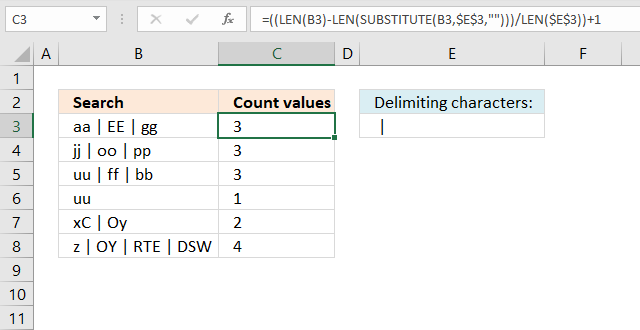The formula in cell C3 uses the characters given in cell E3 to separate and count values in B3. Cell E3 contains " | ", however, you can use whatever characters you want. For example, you can use this formula to separate and count values using a blank as a delimiting character.

Formula in cell C3:

=((LEN(B3)-LEN(SUBSTITUTE(B3,\$E\$3,"")))/LEN(\$E\$3))+1

### 4. How to count the number of values separated by a delimiter - UDF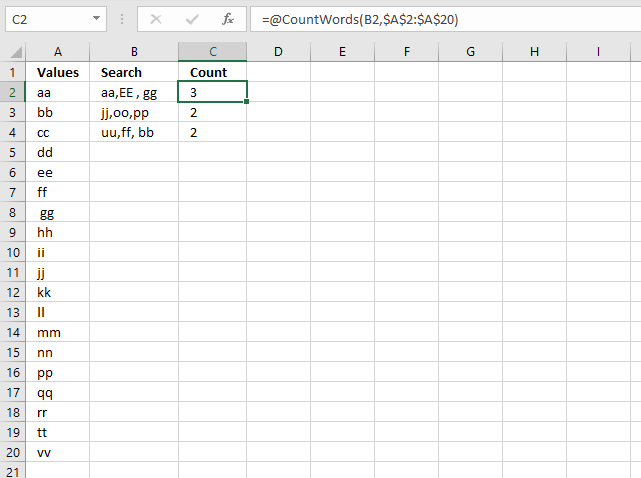I received an email from one of my seven blog readers (joke).

In Excel, I have a column, say A, with some cells blank and some cells with text.

In another column, say B, each *cell* contains many words, separated by a comma.

For every cell in B, I need to check to see how many of the words from column A are in the cell and output the total count of matches.

I built a user-defined function for this, if you have a regular formula you think can solve this, please share. This animated picture explains it all.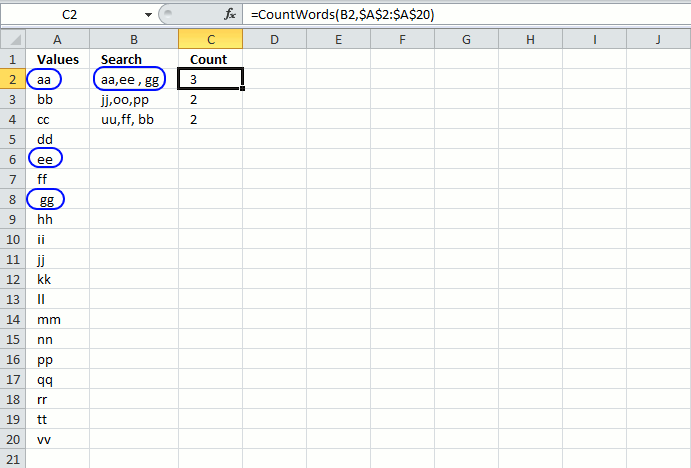The formula in cell C2 is a user defined function. You build a UDF just like a macro using the Visual Basic Editor (VBA).

=CountWords(B2,\$A\$2:\$A\$20)

The first argument (B2) in this custom made function is a cell reference to comma-separated values, in a single cell. The second argument (\$A\$2:\$A\$20) is a reference to cell range that you want to count, make sure it is a single column cell reference.

### 4.1 User-defined function VBA code

```Function CountWords(a As String, b As Range)
Dim Words() As String
Dim Value As Variant, cell As Variant
Dim c As Single
Words = Split(a, ",")
For Each Value In Words
For Each cell In b
If UCase(WorksheetFunction.Trim(cell)) = UCase(WorksheetFunction.Trim(Value)) Then c = c + 1
Next cell
Next Value
CountWords = c
End Function
```

### 4.2 Where to put the VBA code?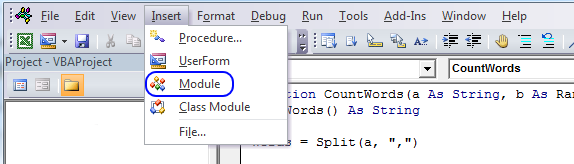To build a user-defined function, follow these steps:

1. Press Alt + F11 to open the visual basic editor.
2. Press with left mouse button on "Insert" on the top menu.
3. Press with left mouse button on "Module", see the image above.
4. Copy the VBA code above and paste it to the code module.

### 4.3 Explaining the user-defined function

Function name and arguments

A user defined function procedure always start with "Function" and then a name. This udf has two arguments, a and b. Variable a is a string and b is a range.

```Function CountWords(a As String, b As Range)
```

Declaring variables

```Dim Words() As String
Dim Value As Variant, cell As Variant
Dim c As Single
```

Words() is a dynamic string array.  Value and cell are variants. c is a single data type. Read more about Defining data types.

Split function

```Words = Split(a, ",")
```

The Split function accepts a text string and returns a zero-based, one-dimensional array containing all sub strings. Split allows you also to specify a delimiting character, default is the space character.

For ... Next statement

```For Each Value In Words
...
Next Value
```

Repeats a group of statements a specified number of times. In this case as many times as there are values in the array Words.

If function

```If UCase(WorksheetFunction.Trim(cell)) = UCase(WorksheetFunction.Trim(Value)) Then c = c + 1
```

The Ucase function converts a string to uppercase letters. The WorksheetFunction.Trim method removes all spaces from text except for single spaces between words.
The If function compares cell and Value and if they match 1 is added to c.

The udf returns...

```CountWords = c
```

The udf returns the value in c.

End a udf

```End Function
```

A function procedure ends with the "End function" statement.

### Get the Excel file